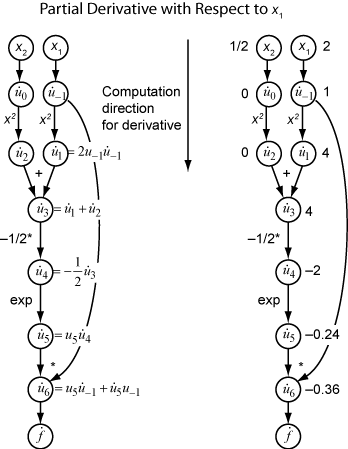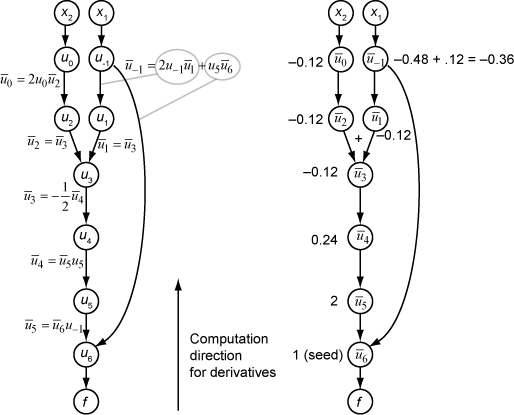Main Content

## Automatic Differentiation Background

### What Is Automatic Differentiation?

Automatic differentiation (also known as autodiff, AD, or algorithmic differentiation) is a widely used tool for deep learning. See Books on Automatic Differentiation. It is particularly useful for creating and training complex deep learning models without needing to compute derivatives manually for optimization. For examples showing how to create and customize deep learning models, training loops, and loss functions, see Define Custom Training Loops, Loss Functions, and Networks.

Automatic differentiation is a set of techniques for evaluating derivatives (gradients) numerically. The method uses symbolic rules for differentiation, which are more accurate than finite difference approximations. Unlike a purely symbolic approach, automatic differentiation evaluates expressions numerically early in the computations, rather than carrying out large symbolic computations. In other words, automatic differentiation evaluates derivatives at particular numeric values; it does not construct symbolic expressions for derivatives.

• Forward mode evaluates a numerical derivative by performing elementary derivative operations concurrently with the operations of evaluating the function itself. As detailed in the next section, the software performs these computations on a computational graph.

• Reverse mode automatic differentiation uses an extension of the forward mode computational graph to enable the computation of a gradient by a reverse traversal of the graph. As the software runs the code to compute the function and its derivative, it records operations in a data structure called a trace.

As many researchers have noted (for example, Baydin, Pearlmutter, Radul, and Siskind ), for a scalar function of many variables, reverse mode calculates the gradient more efficiently than forward mode. Because a deep learning loss function is a scalar function of all the weights, Deep Learning Toolbox™ automatic differentiation uses reverse mode.

### Forward Mode

Consider the problem of evaluating this function and its gradient:

`$f\left(x\right)={x}_{1}\mathrm{exp}\left(-\frac{1}{2}\left({x}_{1}^{2}+{x}_{2}^{2}\right)\right).$`

Automatic differentiation works at particular points. In this case, take x1 = 2, x2 = 1/2.

The following computational graph encodes the calculation of the function f(x).To compute the gradient of f(x) using forward mode, you compute the same graph in the same direction, but modify the computation based on the elementary rules of differentiation. To further simplify the calculation, you fill in the value of the derivative of each subexpression ui as you go. To compute the entire gradient, you must traverse the graph twice, once for the partial derivative with respect to each independent variable. Each subexpression in the chain rule has a numeric value, so the entire expression has the same sort of evaluation graph as the function itself.

The computation is a repeated application of the chain rule. In this example, the derivative of f with respect to x1 expands to this expression:

`$\begin{array}{c}\frac{df}{d{x}_{1}}=\frac{d{u}_{6}}{d{x}_{1}}\\ =\frac{\partial {u}_{6}}{\partial {u}_{-1}}+\frac{\partial {u}_{6}}{\partial {u}_{5}}\frac{\partial {u}_{5}}{\partial {x}_{1}}\\ =\frac{\partial {u}_{6}}{\partial {u}_{-1}}+\frac{\partial {u}_{6}}{\partial {u}_{5}}\frac{\partial {u}_{5}}{\partial {u}_{4}}\frac{\partial {u}_{4}}{\partial {x}_{1}}\\ =\frac{\partial {u}_{6}}{\partial {u}_{-1}}+\frac{\partial {u}_{6}}{\partial {u}_{5}}\frac{\partial {u}_{5}}{\partial {u}_{4}}\frac{\partial {u}_{4}}{\partial {u}_{3}}\frac{\partial {u}_{3}}{\partial {x}_{1}}\\ =\frac{\partial {u}_{6}}{\partial {u}_{-1}}+\frac{\partial {u}_{6}}{\partial {u}_{5}}\frac{\partial {u}_{5}}{\partial {u}_{4}}\frac{\partial {u}_{4}}{\partial {u}_{3}}\frac{\partial {u}_{3}}{\partial {u}_{1}}\frac{\partial {u}_{1}}{\partial {x}_{1}}.\end{array}$`

Let ${\stackrel{˙}{u}}_{i}$ represent the derivative of the expression ui with respect to x1. Using the evaluated values of the ui from the function evaluation, you compute the partial derivative of f with respect to x1 as shown in the following figure. Notice that all the values of the ${\stackrel{˙}{u}}_{i}$ become available as you traverse the graph from top to bottom.To compute the partial derivative with respect to x2, you traverse a similar computational graph. Therefore, when you compute the gradient of the function, the number of graph traversals is the same as the number of variables. This process is too slow for typical deep learning applications, which have thousands or millions of variables.

### Reverse Mode

Reverse mode uses one forward traversal of a computational graph to set up the trace. Then it computes the entire gradient of the function in one traversal of the graph in the opposite direction. For deep learning applications, this mode is far more efficient.

The theory behind reverse mode is also based on the chain rule, along with associated adjoint variables denoted with an overbar. The adjoint variable for ui is

`${\overline{u}}_{i}=\frac{\partial f}{\partial {u}_{i}}.$`

In terms of the computational graph, each outgoing arrow from a variable contributes to the corresponding adjoint variable by its term in the chain rule. For example, the variable u–1 has outgoing arrows to two variables, u1 and u6. The graph has the associated equation

`$\begin{array}{c}\frac{\partial f}{\partial {u}_{-1}}=\frac{\partial f}{\partial {u}_{1}}\frac{\partial {u}_{1}}{\partial {u}_{-1}}+\frac{\partial f}{\partial {u}_{6}}\frac{\partial {u}_{6}}{\partial {u}_{-1}}\\ ={\overline{u}}_{1}\frac{\partial {u}_{1}}{\partial {u}_{-1}}+{\overline{u}}_{6}\frac{\partial {u}_{6}}{\partial {u}_{-1}}.\end{array}$`

In this calculation, recalling that ${u}_{1}={u}_{-1}^{2}$ and u6 = u5u–1, you obtain

`${\overline{u}}_{-1}={\overline{u}}_{1}2{u}_{-1}+{\overline{u}}_{6}{u}_{5}.$`

During the forward traversal of the graph, the software calculates the intermediate variables ui. During the reverse traversal, starting from the seed value ${\overline{u}}_{6}=\frac{\partial f}{\partial f}=1$, the reverse mode computation obtains the adjoint values for all variables. Therefore, the reverse mode computes the gradient in just one computation, saving a great deal of time compared to forward mode.

The following figure shows the computation of the gradient in reverse mode for the function

`$f\left(x\right)={x}_{1}\mathrm{exp}\left(-\frac{1}{2}\left({x}_{1}^{2}+{x}_{2}^{2}\right)\right).$`

Again, the computation takes x1 = 2, x2 = 1/2. The reverse mode computation relies on the ui values that are obtained during the computation of the function in the original computational graph. In the right portion of the figure, the computed values of the adjoint variables appear next to the adjoint variable names, using the formulas from the left portion of the figure.The final gradient values appear as ${\overline{u}}_{0}=\frac{\partial f}{\partial {u}_{0}}=\frac{\partial f}{\partial {x}_{2}}$ and ${\overline{u}}_{-1}=\frac{\partial f}{\partial {u}_{-1}}=\frac{\partial f}{\partial {x}_{1}}$.

For more details, see Baydin, Pearlmutter, Radul, and Siskind  or the Wikipedia article on automatic differentiation .

 Baydin, A. G., B. A. Pearlmutter, A. A. Radul, and J. M. Siskind. "Automatic Differentiation in Machine Learning: a Survey." The Journal of Machine Learning Research, 18(153), 2018, pp. 1–43. Available at https://arxiv.org/abs/1502.05767.

 Automatic differentiation. Wikipedia. Available at https://en.wikipedia.org/wiki/Automatic_differentiation.

Download ebook